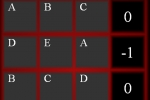Squares Of Doom Subtraction

 Squares Of Doom SubtractionGray - Open, Black - Total.Hover over a box. Type a number.Box A = Box A, Box B = Box B, and so on...Highest possible number is the current level.Have fun!

Tags:
squares    doom    subtraction square    doom    amp    math numbers    amp    math